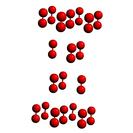Crystal Lattice Structures: Creation Date: 20 Apr 2002 Last Modified: 21 Oct 2004

# The βO2 StructureYou can now

• This structure has the same symmetry and Wyckoff positions as rhombohedral graphite and αAs (A7). The relative positions of the atoms are rather different, however.
• The rhombohedral lattice can be specified by the length of the primitive vectors, a, and the angle between them, θ, or by the lattice constants of the equivalent hexagonal lattice. In terms of a and θ we have

ahex = 2 a sin(½ θ)

chex = a {3 [1 + 2 cos(θ)]}½

• Prototype: βO2
• Pearson Symbol: hR2
• Space Group: R3m (Cartesian and lattice coordinate listings available)
• Number: 166
• Reference: R. J. Meier and R. B. Helmholdt, Phys. Rev. B 29, 1387 (1984)
• Primitive Vectors:  A1 = + ahex/2 X - ahex/(2 31/2) Y + chex/3 Z A2 = + ahex/31/2 Y + chex/3 Z A3 = - ahex/2 X - ahex/(2 31/2) Y + chex/3 Z
• Basis Vectors:  B1 = + z (A1 + A2 + A3) = + z chex Z (O) (2c) B2 = - z (A1 + A2 + A3) = - z chex Z (O) (2c)

Go back to the III-VII elements page.

Go back to Crystal Lattice Structure page.

 Structures indexed by: This is a mirror of an old page created at theNaval Research LaboratoryCenter for Computational Materials ScienceThe maintained successor is hosted at http://www.aflowlib.org/CrystalDatabase/ and published as M. Mehl et al., Comput. Mater. Sci. 136 (Supp.), S1-S828 (2017).